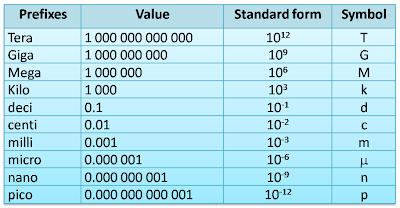# Prefixes

Prefixes are the preceding factor used to represent very small and very large physical quantities in SI units.

Table below shows the prefixes that you need to know in SPM.### Conversion of prefixes

#### Prefixes to Normal Number

Example 1:
The frequency of the radio wave is 350M Hz. What is the frequency of the radio wave in Hz?

Mega (M) = 1,000,000 or 106

Therefore,
350MHz = 350 x 106Hz

Example 2:
The thickness of a film is 25nm. What is the thickness in unit meter?

nano (n) = 0.000000001 or 10-9

Therefore
25nm = 25 x 10-9m

#### Normal number to Prefixes

Example 3:
0.255 s is equal to how many ms .